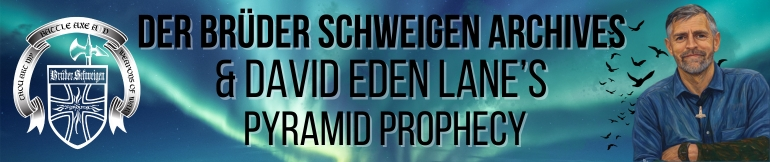Mystery Religions and the Seven Seals "Chapter Four - 666 Unveiled" Introduction - Chapter 1 - Coding - Chapter Two - The Seven Seals - Chapter Three - The Temple - Chapter Four - 666 UNVEILED - Chapter Five - The Mysteries Presented with contempt for the priestcrafters who declare with the infinite arrogance of the ignorant their 666 fantasies. Shown in chapter 2 is an ancient mathematical device used by Secret Societies, Hermetic Philosophers, Occultists and Kabalists for thousands of years. It is called the "Magic 666 Square of the Sun." The Sun Square is comprised of each of the numbers 1 through 36, which, when added together, total 666. The 36 numbers are arranged in 6 rows and 6 columns. The sum of each row, column and diagonal totals 111, or 1/6 of 666. The bible is comprised of 66 books; three of the books have 6 chapters, to encode 666. The book of Isaiah has 66 chapters; three of the chapters have six verses to encode 666. Of the 66 books of the bible, 39 are Old Testament and 27 are New Testament, as 39² + 27² + 66² = 6606, to encode 666. The numerical values assigned to the 12 signs of the Zodiac are the 12 factors of 666. The most basic pattern or function of the Sun Square is the number 74, which is called by Adepts in the Mystery Religions the "Divine number of the Creator. The Sun Square is the 4th of 7 "magic squares. In English language gematria (A=1,B=2,C=3, etc.) we have already seen that the word "GOD" mirrors the Sun Square: G = 7 O = the sun inside the Zodiac D = 4 The 666 Sun Square contains 9 squares and rectangles whose four corners total 74, as 9 x 74 = 666. Concentric Squares: 6 + 1 + 31 + 36 = 74 11 + 8 + 26 + 29 = 74 16 + 15 + 21 + 22 = 74 Rectangles: 7 + 30 + 12 + 25 = 74 19 + 24 + 13 + 18 = 74 32 + 35 + 2 + 5 = 74 3 + 34 + 4 + 33 = 74 14 + 23 + 17 + 20 = 74 27 + 28 + 9 + 10 = 74 The ancient mythology of a Sun God, born at the winter solstice, resurrected near the vernal equinox, worshipped on Sun-day, whose name demonstrates the Kabalistic number canon is a certain sign of a hermetically created Mystery religion.Jesus equals 74. ``` J = 10 E = 5 S = 19 U = 21 S = +19 74``` Just coincidence cry the believers, as their eyes glaze in irrational denial. David Lane